# tensorflow2.0实现鸢尾花数据集的分类(3)——激活函数

#### 1.谜题揭晓

tensorflow2.0实现鸢尾花数据集的分类(2)——神经网络搭建

with tf.GradientTape() as tape:
#前向传播计算 y, y为预测结果
y = tf.matmul(x_train, w) + b
# 计算损失函数
loss = (y - y_train)**2
loss = tf.reduce_mean(loss, axis=0)


$y_1 = x*w_1 + b_1\qquad(1)\\ y_2 = y_1 * w_2 + b_2\qquad(2)$

\begin{aligned} y_2 &= y_1 * w_2 + b_2\\ &= ( x*w_1 + b_1) * w_2 + b_2\\ &=x * (w_1 * w_2) + (b_1 * w_2 + b_2) \end{aligned}

#### 2.激活函数特点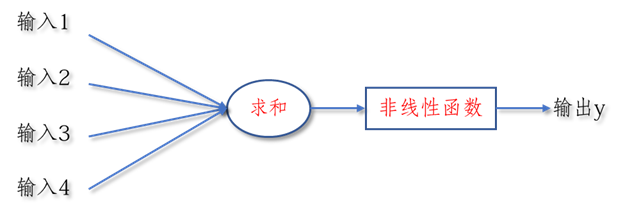• 非线性： 只有激活函数非线性时，才不会被单层网络所替代，多层神经网络可逼近所有函数
• 可微性： 优化器大多用梯度下降更新参数 ，若激活函数不可微，则无法更新参数
• 单调性： 当激活函数是单调的，能保证单层网络的损失函数是凸函数
• 近似恒等性： 有$f(x)\approx x$，当参数初始化为随机小值时，神经网络更稳定

#### 3.常用激活函数介绍

##### (1).$Sigmoid$函数
• 表达式：$f(x)=\frac{1}{1+e^{-x}}$
• tensorflow API ：tf.nn.sigmoid(x)
• 函数图像：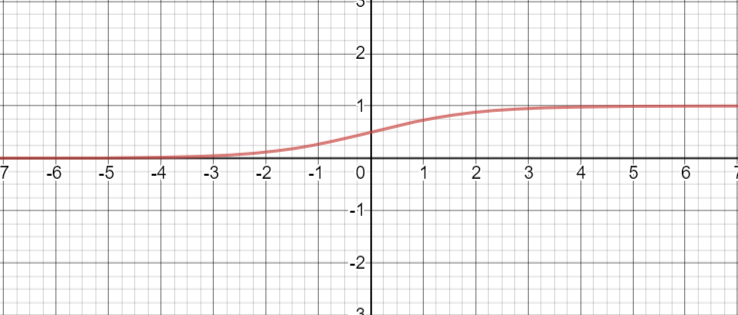• 导数图像：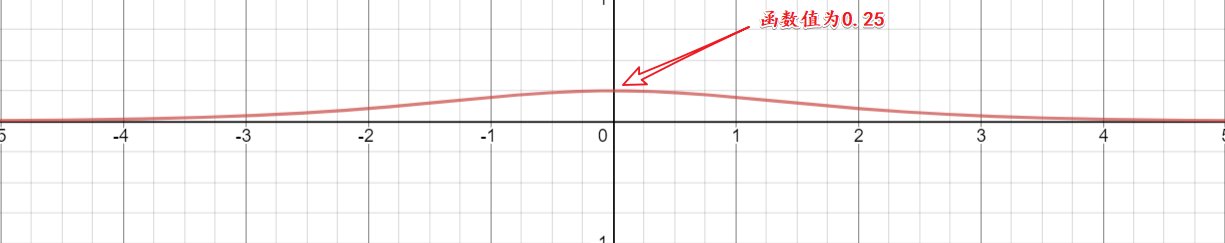• 重要特点：
1. $sigmoid$将输入变为0到1的值，相当于对输入进行了归一化
2. 容易造成梯度消失，因为在更新参数时，需要从输出层到输入层进行链式求导，而我们可以从导数图线看出其导数值的范围是0到0.25，所以在进行链式求导时，需要多个0到0.25的数字相乘，容易造成梯度消失，使得参数无法继续更新(可以看做存在饱和($Saturated$)现象)
3. 输出的均值非0，收敛慢，我们希望输出是以0为均值的，而其输出为0到1使得收敛变慢
4. 存在幂运算，计算复杂度大，训练时间长
##### (2).$Tanh$函数
• 表达式：$f(x)=\frac{1-e^{-2x}}{1+e^{-2x}}$
• tensorflow API ：tf.math.tanh(x)
• 函数图像：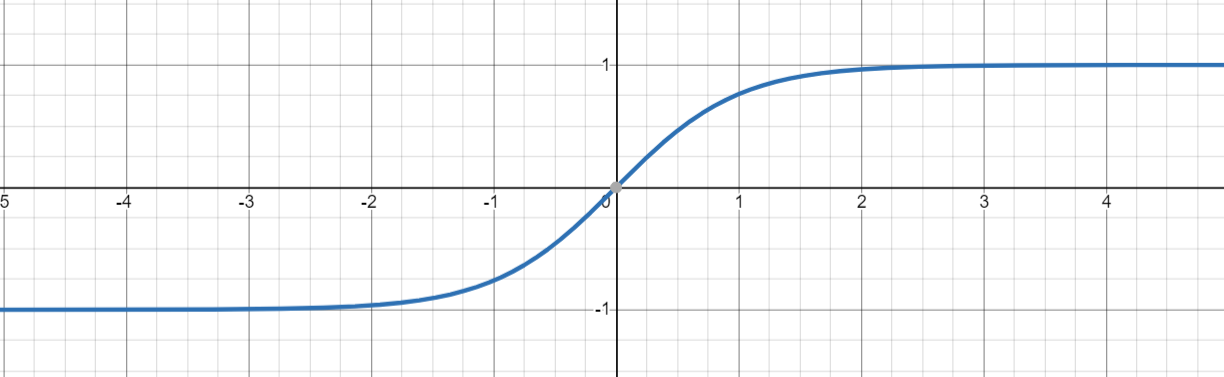• 导数图像：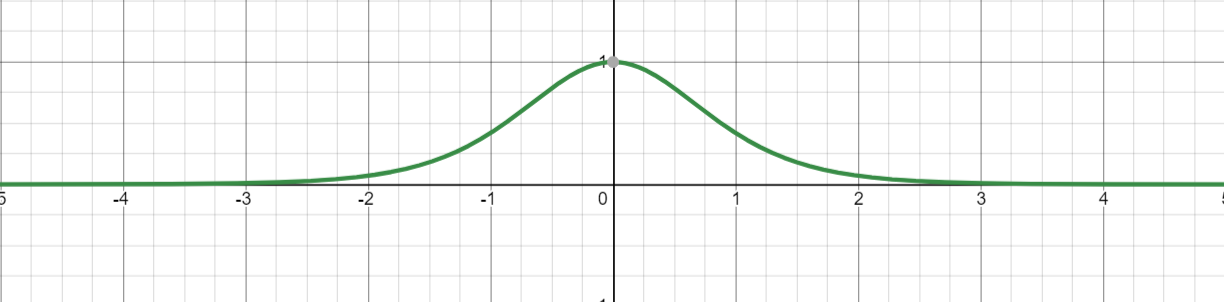• 重要特点：
1. 输出均值为0
2. 仍然易造成梯度消失(可以看做存在饱和现象)
3. 含有幂运算，训练时间长
##### (3).$relu$函数
• 表达式：\begin{aligned} f(x)&=max\{x, 0\}\\ &=\left\{\begin{matrix} x \qquad x\geqslant 0 \\ 0 \qquad x<0 \end{matrix}\right. \end{aligned}
• tensorflow API ：tf.nn.relu(x)
• 函数图像：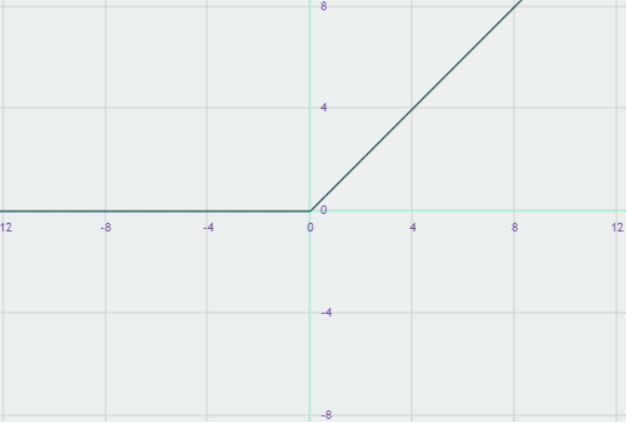• 导数图像：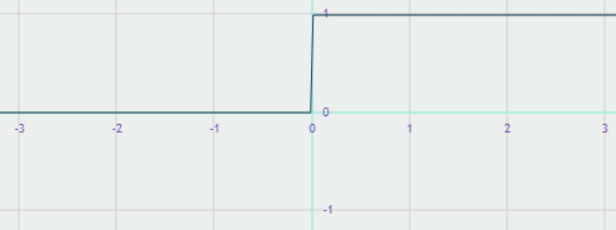• 重要特点：
1. 在正区间内解决了梯度消失问题
2. 计算速度快，只需要解决输入是否大于0
3. 收敛速度快于$sigmoid$$tanh$
4. 输出均值非0
5. 存在 $Dead\ relu$问题，即送入激活函数的特征是负数时，激活函数输出是0，反向传播得到的梯度为0，导致参数无法更新，造成神经元死亡
##### (4).$leaky\ relu$函数
• 表达式：\begin{aligned} f(x)&=max\{x, ax\}\\ &=\left\{\begin{matrix} x \qquad x\geqslant 0 \\ ax \qquad x<0 \end{matrix}\right. \end{aligned}
• tensorflow API ：tf.nn.leaky_relu(x)
• 函数图像：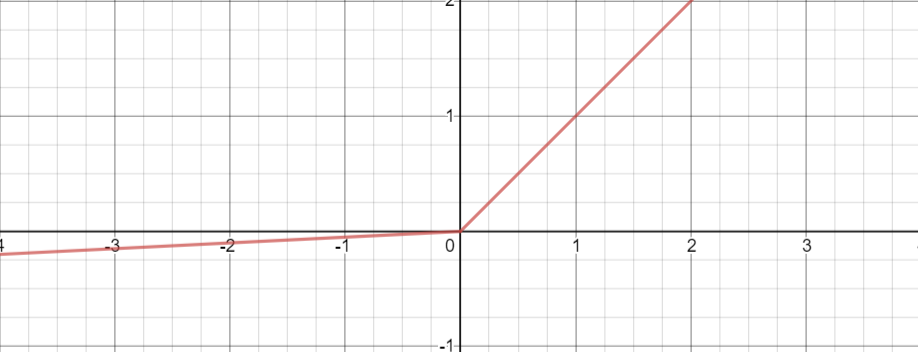-导数图像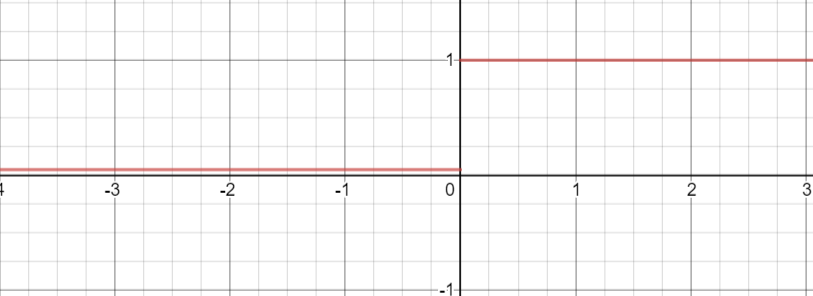• 重要特点：
理论上来讲，$Leaky\ ReLU$$ReLU$的所有优点，外加不会有$Dead\ ReLU$问题，但是在实际操作当 中，并没有完全证明$Leaky\ ReLU$总是好于$ReLU$

#### 4.更改神经网络:

with tf.GradientTape() as tape:
#前向传播计算 y, y为预测结果
y = tf.matmul(x_train, w) + b
y = tf.nn.relu(y)       # <-------------------------在此处加入激活函数即可，没错，就加一句代码
# 计算损失函数
loss = (y - y_train)**2
loss = tf.reduce_mean(loss, axis=0)


#### 5.感谢在线绘图网站:

https://www.desmos.com/calculator

$\{x>0\}\ x\\ \{x<0\}\ 0$

©️2019 CSDN 皮肤主题: 数字20 设计师: CSDN官方博客